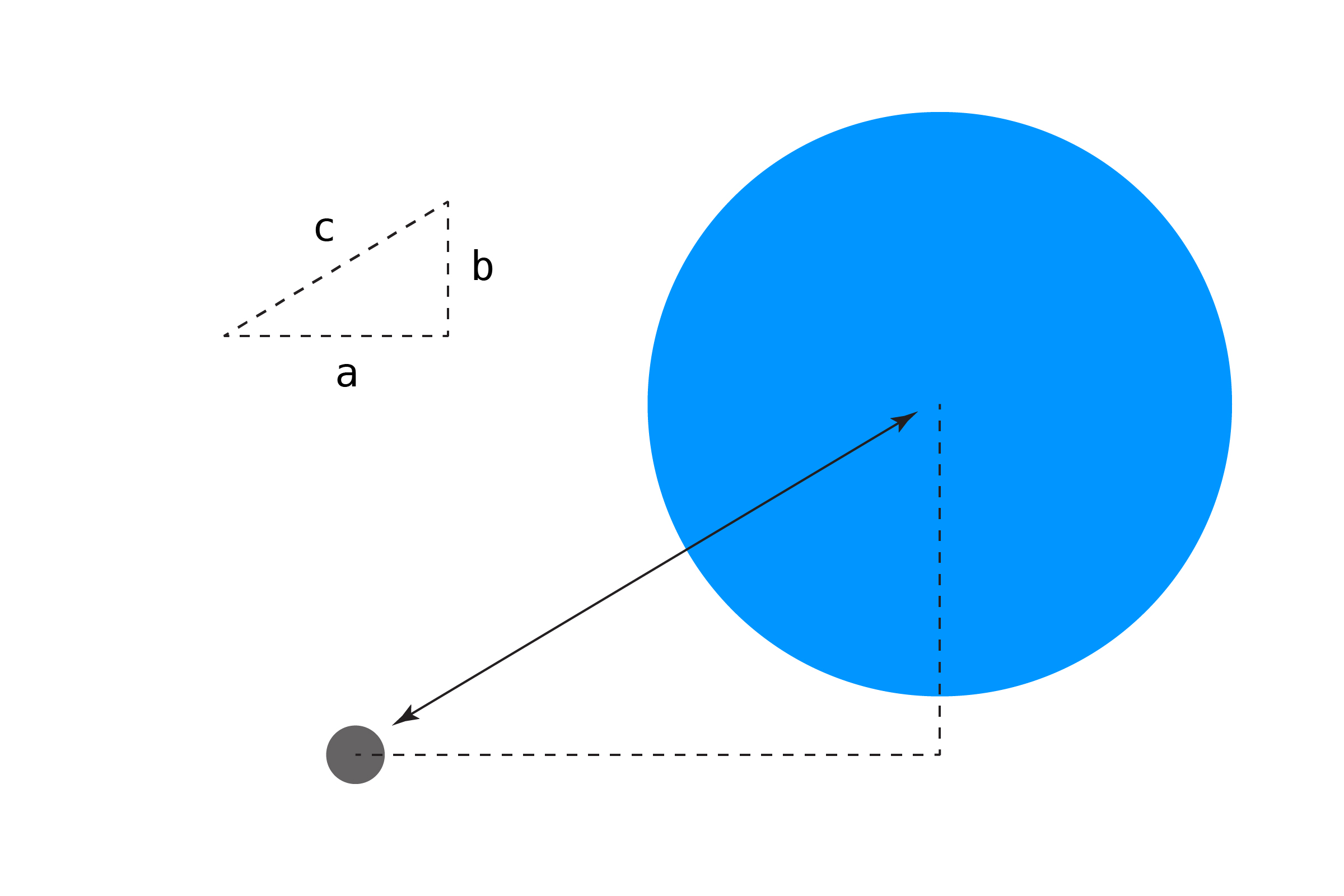[ your browser does not support the canvas tag ]

# POINT/CIRCLE

Point/Point collision was very easy, but from here on out we'll need some basic math to check if objects are touching each other. Testing if a point is inside a circle requires us to remember back to middle school math class and the Pythagorean Theorem:

``````a2 + b2 = c2
``````

We can get the length of the long edge of a triangle `c` given the length of the other two sides. Translated to code, it looks like this:

``````c = sqrt( (a*a) + (b*b) );
``````

Multiply `a` by itself, same for `b`, add the two together, and get the square root of the result.

Why do we need this? We can use the Pythagorean Theorem to get the distance between two objects in 2D space! In this context, `a` and `b` are the horizontal and vertical distances between the point and the center of the circle.We can calculate the X and Y distance like this:

``````float distX = px - cx;
float distY = py - cy;
``````

Then we can find the distance between the two points using the Pythagorean Theorem:

``````float distance = sqrt( (distX*distX) + (distY*distY) );
``````

So if the point is at `(10,10)` and the circle's center is at `(40,50)`, we get a distance of `50`. You might be thinking, "What if the distance comes out negative?" Not to worry: since we multiply the distance values by themselves, even if they are negative the result will be positive.

OK, but how do we use this to test for collision? If the distance between the point and the center of the circle is less than the radius of the circle, we're colliding!

``````if (distance <= r) {
return true;
}
return false;
``````

Used in a full example, we can change the color of the circle if the point is inside it.

``````float px =     0;      // point position
float py =     0;

float cx =     300;    // circle center position
float cy =     200;

void setup() {
size(600,400);
noCursor();

strokeWeight(5);   // thicker stroke = easier to see
}

void draw() {
background(255);

// update point position to mouse coordinates
px = mouseX;
py = mouseY;

// check for collision!
boolean hit = pointCircle(px,py, cx,cy, radius);

// draw circle
// change fill color if hit
if (hit) {
fill(255,150,0);
}
else {
fill(0,150,255);
}
noStroke();

// draw the point
stroke(0);
point(px, py);
}

// POINT/CIRCLE
boolean pointCircle(float px, float py, float cx, float cy, float r) {

// get distance between the point and circle's center
// using the Pythagorean Theorem
float distX = px - cx;
float distY = py - cy;
float distance = sqrt( (distX*distX) + (distY*distY) );

// if the distance is less than the circle's
// radius the point is inside!
if (distance <= r) {
return true;
}
return false;
}
``````

This method using the Pythagorean Theorem will come back many times. Processing has a built-in `dist()` function, if you prefer, though we'll keep the math in place as a reference.

One caveat: if you have a very fast-moving object, it can sometimes go right through its target without a collision being triggered! This is sometimes referred to as the "bullet through paper" problem. There are lots of solutions, but a good place to start would be this GameDev.net post. A standard way for detecting this is called "Continuous Collision Detection" or CCD.

NEXT: Circle/Circle